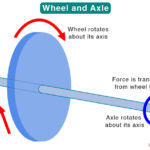Home / Physics / Types of Forces

# Types of Forces

## What is a Force

A force is a push or a pull acting on an object due to its interaction with another object. It causes a stationary object to move and a moving object to come to a stop. Force is a vector quantity that has both magnitude and direction.

## Force Equation

How to Find Force

The force equation can be derived by using Newton’s Second Law, which states that the force (F) acting on an object is given by the product of its mass (m) and acceleration (a).

F = ma

Acceleration is defined as velocity (v) over time (t).

F = mv/t

SI Unit of Force: Newtons or N (kg.m.s-2)

Dimension of Force: [MLT-2]

## Characteristics of a Force

Here are some facts, characteristics, and properties of a force.

• Defined as the interaction between two objects
• Effect is to change the motion (magnitude and direction) of an object
• Effect is to change the shape of an object
• Vector quantity – Resultant force on an object is the vector sum, which gives rise to balanced force and unbalanced force.

## Classification of Forces

Generally, forces can be classified into two types based on their application. They are contact and non-contact forces.

### Contact Force

A contact force is defined as the force between two objects that are in physical contact. Here are some types of contact force.

#### 1. Normal Force

When an object lies on a surface, the surface exerts an equal and opposite force.  The component of this force that is perpendicular to the surface is called the normal force.

Example: The force exerted by the table on a book lying on it.

#### 2. Applied Force

The forces applied to an object in order to displace or deform it is called the applied force.

Example: The force with which a person pushes a cart.

#### 3. Tension Force

The tension or tension force is a force experienced by a rope, string, or cable pulled from the opposite end.

Example: The force experienced by a rope when a person pulls a bucket of water from a well.

#### 4. Friction

The friction force is the force of resistance to an object’s motion. There are two main types of friction force.

##### A. Static Friction

Static friction arises when the applied force is such that an object remains stationary relative to the surface.

Example: The resistance offered by a stationary box when a person tries to push it.

##### B. Kinetic Friction

Kinetic friction arises when the applied force is such that it displaces an object, which then moves relative to the surface. Kinetic friction is of two types.

1. Sliding Friction: It is the resistance offered by the surface when the object is sliding

Example: The resistance force offered by the floor when a person pushes a box over it.

2. Rolling Friction: It is the resistance offered by the surface when the object is rolling

Example: The resistance offered by the floor when a person pushes a cart over it.

There is another type of friction called fluid friction. It is the resistance to an object’s motion in a fluid.

Example: A person swimming in the water.

#### 5. Air Resistance

The force of resistance offered by air to an object moving through it is called air resistance.

Example: The resistance offered by air when a ball falls through it.

#### 6. Spring Force

The force exerted by a spring when compressed or extended is called spring force.

Example: The force used to measure weight with a weighing scale.

#### 7. Buoyant Force

When an object is immersed in a fluid, wholly or partially, the fluid exerts an upward force opposite its weight. This phenomenon is known as buoyancy, and the upward thrust is known as the buoyant force.

Example: Boat sailing on the river.

#### 8. Drag Force

When an object moves through a fluid, like air and water, it experiences a drag force or drag resistance.

Example: A car driving on a highway.

### Non-contact Force

A non-contact force is defined as the force between two objects that are not in physical contact. It is also called a field force. Here are the different types of non-contact forces.

#### 1. Gravitational Force and Gravity

The force of attraction between astronomical objects is called the gravitational force. The force of attraction between Earth and any other object on its surface is called gravity. The force due to gravity can be measured using a spring balance.

Example: (Gravitational Force) The force of attraction between the Sun and the Earth. (Gravity) The force experienced by a person standing on the surface of the Earth.

#### 2. Electrostatic Force

The electrostatic force is the force of attraction or repulsion between two charged particles. It is also called Coulomb’s force or Coulomb’s interaction.

Example:The force with which electrons are held together by the nucleus.

#### 3. Magnetic Force

The force experienced by an object in the presence of a magnet is called magnetic force.

Example: The force of attraction between a bar magnet and an iron nail.

## FAQ

Q.1 What the four main types of resistance forces?

Ans. The four main types of resistance forces are friction, gravity, air resistance, and buoyancy.

Q.2. What are the four types of fundamental forces of nature?

Ans. The four fundamental forces of nature are gravitational force, electromagnetic force, strong nuclear force, and weak nuclear force.

Article was last reviewed on Thursday, June 24, 2021

### Related articlesWheel and Axle Simple MachinePulley Simple MachineWedge Simple MachineScrew Simple Machine